The NLMIXED Procedure

Hessian Scaling

The rows and columns of the Hessian matrix can be scaled when you use the trust region, Newton-Raphson, and double-dogleg optimization techniques. Each element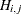,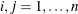is divided by the scaling factor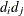, where the scaling vector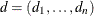is iteratively updated in a way specified by the HESCAL= i option, as follows: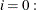No scaling is done (equivalent to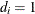).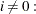First iteration and each restart iteration sets: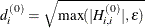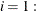See Moré (1978):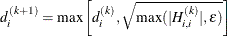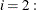See Dennis, Gay, and Welsch (1981):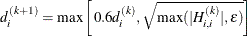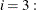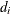is reset in each iteration: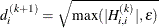In the preceding equations,is the relative machine precision or, equivalently, the largest double-precision value that, when added to 1, results in 1.